Courses

# Friction Force MCQ Level - 2

## 10 Questions MCQ Test Basic Physics for IIT JAM | Friction Force MCQ Level - 2

Description
This mock test of Friction Force MCQ Level - 2 for Physics helps you for every Physics entrance exam. This contains 10 Multiple Choice Questions for Physics Friction Force MCQ Level - 2 (mcq) to study with solutions a complete question bank. The solved questions answers in this Friction Force MCQ Level - 2 quiz give you a good mix of easy questions and tough questions. Physics students definitely take this Friction Force MCQ Level - 2 exercise for a better result in the exam. You can find other Friction Force MCQ Level - 2 extra questions, long questions & short questions for Physics on EduRev as well by searching above.
QUESTION: 1

### A box of mass 8kg is placed on a rough inclined plane of inclination θ. Its downward motion can be prevented by applying an upward pull F and it can be made to slide upwards by applying a force 2F. The coefficient of friction between the box and the inclined plane is

Solution:

During downward motion :
F = mg Sinθ - mg Cosθ
During upward motion :
2F = mg Sinθ + μmg Cosθ
Solving above two equations, we get m = 1/3 tanθ
The correct answer is: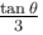QUESTION: 2

### The coefficient of friction between 4kg and 5kg blocks is 0.2 and between 5kg blocks and ground is 0.1 respectively. Choose the correct statement :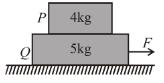Solution:

So block 'Q' is moving due to force while block 'P' due to friction.
Friction direction on both P + Q blocks as shown.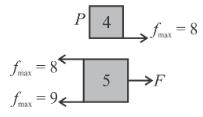First block 'Q' will move and P will move with 'Q' so by FBD taking 'P' and 'Q' as system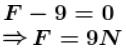When applied force is 4 N  then FBD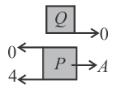i.e., ap = aq = 0
kg block is moving due to friction and maximum friction force is 8 N
So acceleration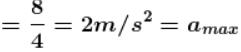Slipping will start at when Q has +ve acceleration equal to maximum acceleration of P i.e., 2 m/s2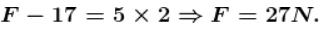The correct answer is: Maximum acceleration of 4 kg block is 2 m/s2

QUESTION: 3

### A block of mass 1kg lies on a horizontal surface in a truck. The coefficient of static friction between the block and the surface is 0.6. If the acceleration of the truck is 5 m/s2, the friction force acting on the block is :

Solution:

Maximum friction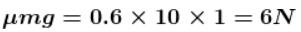Pseudo force = ma = 5N
acceleration due to the applied Pseudo force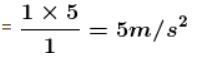So ​required friction force is only 5N although maximum friction force available is 6N.
The correct answer is: 5 N

QUESTION: 4

In the following figure, find the direction of friction on the blocks and ground respectively.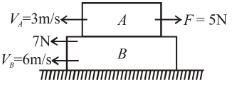Solution:

Direction of friction is such that it opposes the relative velocity.
The correct answer is: Block A (left), block B (right due to block A, right due to ground)

QUESTION: 5

A chain of length L is placed on a horizontal surface as shown in figure. At any instant x is the length of chain on rough surface and the remaining portion lies on smooth surface. Initially x = 0. A horizontal force P is applied to the chain (as shown in the figure). In the duration x changes from x = 0 to x = L, for chain to move with constant speed :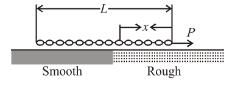Solution:

For chain to move with constant speed P needs to be equal to frictional forces on the chain. As the length of chain on the rough surface increases.
Hence the friction force fk = µkincreases.
The correct answer is: the magnitude of P should increase with time

QUESTION: 6

In the arrangement shown in the figure mass of the block B and A are 2m, 8m respectively. Surface between B and floor is smooth. The block B is connected to block C by means of a pulley. If the whole system is released then the minimum value of mass of the block C so that the block A remains stationary with respect to B is :

(Co-efficient of friction between A and B is μ).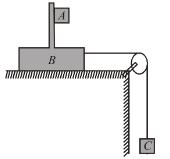Solution:

FBD of A and C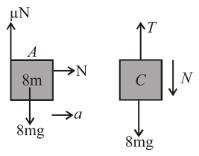If acceleration of 'C' is a
For block 'A' N = 8 ma ...(1)
for block A to remain stationary with respect to block B,
8mg = μN (Limiting condition)   ...(2)
a = g/μ
and acceleration a can be written by the equation of system (A + B + C)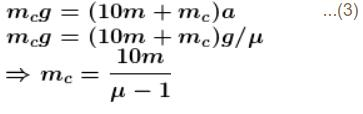The correct answer is: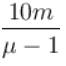QUESTION: 7

The two blocks, m = 10kg and M = 50kg are free to move as shown. The coefficient of static friction between the blocks is 0.5 and there is no friction between M and the ground. A minimum horizontal force F is applied to hold m against M that is equal to :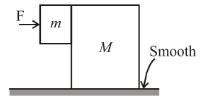Solution:

Limiting condition for m to not slip in vertical downward direction,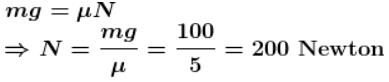Same normal force would acceleration M, thus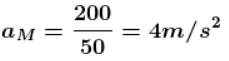Taking m + M as system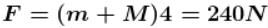The correct answer is: 240 N

QUESTION: 8

A given object takes n times more time to slide down 45° rough inclined plane as it takes to slide down a perfectly smooth 45° incline. The coefficient of kinetic friction between the objects and the incline is.

Solution: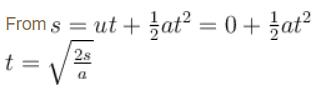For smooth plane  a = g sinθ
For rough plane, a' = g(sinθ - μcosθ)
∴ t' = nt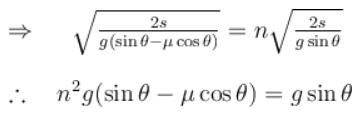when θ = 45° ,  sinθ = cosθ = 1/√2
On solving we get,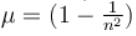The correct answer is: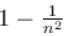QUESTION: 9

A force F = t is applied to a block A as shown in figure, where t is time in seconds. The force is applied at t = 0 seconds when the system was at rest. Which of the following graph correctly give the frictional force between A and horizontal surface as a function of time t. [Assume that at t = 0, tension in the string connecting the two blocks is zero].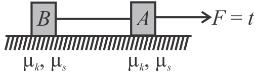Solution:

Let mA and mB be the mass of blocks A and B respectively.
As the force F increases from 0 to µs mAg, the frictional force f on block A is such that f = F. When F = µsmAg, the frictional force f attains maximum value f = µsmg.
As F is further increased to µs(mA + mB)g, the block A does not move. In this duration frictional force on block A remains constant at µsmAg.
As F further increased, system will start moving and kinetic friction (µxAg) will start acting on A (µsk). Hence C is correct choice.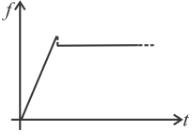QUESTION: 10

Given mA = 20kgmB = 10kgmC = 20kg. Between A and B, µ1 = 0.3, between B and C, µ2 = 0.3 and between C and ground µ3 = 0.1. The least horizontal force F to start the motion of any part of the system of three blocks resting upon one another as shown in figure is (g = 10 m/s2)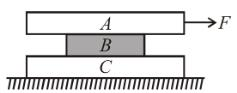Solution:

Limiting friction between A and B = 60 N
Limiting friction between B and C = 90 N
Limiting friction between C and ground = 50 N
Since limiting friction is least between C and ground, slipping will occur at first between C and ground. This will occur when F = 50 N.
The correct answer is: 60 N